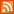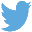Bucaro TecHelpHTTPS Encryption not required because no account numbers orpersonal information is ever requested or accepted by this siteCustom Search# JavaScript Code for Binary to Decimal - Decimal to Binary Conversion

In the number systems in use today, the digits are arranged in succession, the position of each digit has a place value. Positional notation means that the value of a digit in a number depends on its location in the number. The values of the digits increases from right-to-left.

 Digit Number 6 5 4 3 2 1 0 Exponent 106 105 104 103 102 101 100 Value 1000000 100000 10000 1000 100 10 1

The decimal system is a base 10 system. In a base 10 system, the number in the farthest right position indicates the number of 1s. The next position to the left indicates the number of 10s. The next position to the left of the 10s indicates the number of 100s, and so on, raising the value of each position by the positions location number used as the exponent in a power-of-ten.

 Bit Number 7 6 5 4 3 2 1 0 Exponent 27 26 25 24 23 22 21 20 Value 128 64 32 16 8 4 2 1

Binary numbers use a similar positional notation, with the farthest right position indicating the number of 1s. The next position to the left indicates the number of 2s (10s in binary). The next position to the left of the 10s indicates the number of 4s (100s in binary), and so on, raising the value of each position by the positions location number used as the exponent in a power-of-two.

To convert a binary number to decimal, if the position contains a 1, add that positions value, in decimal, to the value of the decimal number. Continue adding the decimal values of all the positions containing a 1. Shown below is JavaScript to convert the binary number 1001011 to decimal.

```var dec = 0;
var inbin = "1001011";
var bits = inbin.split('');
var inbinRev = bits.reverse();

var last = inbin.length;

for(var i = 0; i < last; i++)
{
if(inbinRev[i] == "1")
{
dec += Math.pow(2,i);
}
}

```

In operation, the string split method is used split the binary number string into an array of characters, in this case returning ["1","0","0","1","0","1","1"]. Then the array reverse method is used to put the bits in order of increasing value. This just makes the code easier to understand.

Then a for loop is used to run through the bits and test if a bit is a "1". If a bit is a "1", using the Math pow method, the positional value of the bit is calculated and added to the decimal value held in the variable dec.

Now, I know there are all kinds of other methods to convert a binary number to a decimal number, some may be more efficient than this one. But this is a more pedagogical method.

Decimal to Binary Conversion

To perform decimal to binary conversion, just do the opposite of the above. Look for the greatest power of 2 that will fit into the decimal number. Subtract the decimal value of that power of 2 from the decimal number and set the most significant position of the binary number to 1.

 .menubtn { margin-top:10px; margin-left:50px; width:220px; opacity:1; border-style:solid; border-color:#009000; background-color:#66ff66; transition-property: opacity; transition-duration: 1s; transition-timing-function: ease-out; } .menubtn:hover { opacity:1; background-color:#e5ff23; } Menu - More Java Script ProgrammingRSS FeedFollow @Stephen Bucaro[Site User Agreement] [Privacy Policy] [Site map] [Search This Site] [Contact Form]
Copyright©2001-2023 Bucaro TecHelp 13771 N Fountain Hills Blvd Suite 114-248 Fountain Hills, AZ 85268